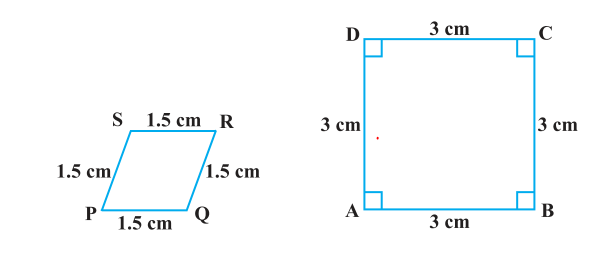# NCERT Solutions for Class 10 Maths: Chapter 6 Similar triangles Exercise 6.1

## Triangles EXERCISE 6.1

In this page we have NCERT Solutions for Class 10 Maths: Chapter 6 Similar triangles for Exercise 6.1 on page 122. Hope you like them and do not forget to like , social_share and comment at the end of the page.
Concept/Formula Used
Two polygons of the same number of sides are similar, if (i) all the corresponding angles are equal and (ii) all the corresponding sides are in the same ratio (or proportion).
Question 1.
Fill in the blanks using correct word given in the brackets: -

(i) All circles are __________. (congruent, similar)
(ii) All squares are __________. (similar, congruent)
(iii) All __________ triangles are similar. (isosceles, equilateral)
(iv) Two polygons of the same number of sides are similar, if (a) their corresponding angles are __________ and (b) their corresponding sides are __________. (equal, proportional)
(i) Similar
(ii) Similar
(iii)Equilateral
(iv) (a) Equal (b) Proportional
Question 2.
Give two different examples of pair of
(i) Similar figures
(ii) Non-similar figures
(i) Examples of Similar figures would be (a)Two equilateral triangles of sides 2 cm and 6 cm respectively
(b)Two Square of sides 2 and 6 cm respectively
(ii)Examples of non-Similar figures would be a) Square and a circle
b) Triangle and square

Question 3.
State whether the following quadrilaterals are similar or not:Answer
We know that two polygons are similar if (i) their corresponding angles are equal and (ii) their corresponding sides are in the same ratio. Here the given two figures have corresponding sides in the same ratio but the corresponding angles are not equal. So, these figures are not similar

## Summary

1. NCERT Solutions for Class 10 Maths: Chapter 6 triangles Exercise 6.1 has been prepared by Expert with utmost care. If you find any mistake.Please do provide feedback on mail.You can download this as pdf
2. This chapter 6 has total 5 Exercise 6.1 ,6.2,6.3 ,6.4 and 6.5. This is the First exercise in the chapter.You can explore previous exercise of this chapter by clicking the link belowGo back to Class 10 Main Page using below links

### Practice Question

Question 1 What is $1 - \sqrt {3}$ ?
A) Non terminating repeating
B) Non terminating non repeating
C) Terminating
D) None of the above
Question 2 The volume of the largest right circular cone that can be cut out from a cube of edge 4.2 cm is?
A) 19.4 cm3
B) 12 cm3
C) 78.6 cm3
D) 58.2 cm3
Question 3 The sum of the first three terms of an AP is 33. If the product of the first and the third term exceeds the second term by 29, the AP is ?
A) 2 ,21,11
B) 1,10,19
C) -1 ,8,17
D) 2 ,11,20